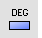# ChangeDegreeCurve ToolsSurface Tools

Edit

Change Degree

The ChangeDegree command changes the degree of the polynomial that defines the curve or surface by adding or subtracting control points between knot spans, while maintaining the knot structure.

#### Steps

1. Select the objects.
2. Type a degree value.
For surfaces type values in the u and v directions.
Command-line options

Deformable

Yes

If the degree of the curve/surface is different from the requested degree, the new curve/surface will have simple interior knots.

##### No

If the degree of the curve/surface is LESS than the requested degree, the new curve/surface will have exactly the same geometry and parameterization as the original, but it will have knots of multiplicity = old multiplicity + new degree - old degree.

If the degree of the curve/surface is GREATER than the requested degree, the new curve/surface will be an approximation of the original with simple knots.

Type subcrv to select part of a curve as input.

#### Note

• The ChangeDegree command changes the degree of the polynomial Rhino uses to create the curve. It keeps the knot structure of the curves the same, but it adds or subtracts control points between each knot span.
• Often it is better to use InsertKnot and RemoveKnot instead of ChangeDegree, because you can add/remove control points more locally.
• To make the surface have the same geometry, but more control points for editing, increase the degree to 5 with the Deformable option set to No. In this case, consider using the InsertKnot command, which will add control points but leave the degree unchanged.
• When exporting geometry to another application, make every attempt to keep the degree of your surfaces low. In particular, many mechanical CAD systems cannot import surfaces that have degrees greater than 3.
• The number of control points added or subtracted depends on the degree; the higher the degree, the greater the number of control points.
• If the degree of a surface is raised with Deformable=No, the surface will no longer be periodic. Use MakePeriodic to restore the periodicity of the surface.
• Objects with higher degrees take longer to display and use more memory.